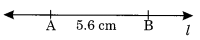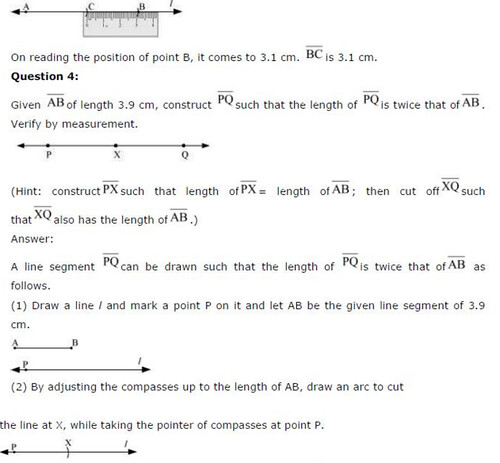# NCERT Solutions For Class 6 Maths Practical Geometry Exercise 14.2

NCERT Solutions For Class 6 Maths Practical Geometry Exercise 14.2

### NCERT Solutions For Class 6 Maths Practical Geometry Exercise 14.2

NCERT Solutions For Class 6 Maths Chapter 14 Practical Geometry Ex 14.2

Exercise 14.2

Ex 14.2 Class 6 Maths Question 1.
Draw a line segment of length 7.3 cm using ruler.
Solution:
Step I: Mark at point P.
Step II : Place the O mark of the ruler against the point P.
Step III : Mark a point Q at a distance of 7.3 cm from P.
Step IV : Join P and Q.Ex 14.2 Class 6 Maths Question 2.
Construct a line segment of length 5.6 cm using ruler and compass.
Solution:
Step I: Draw any line L of suitable lengths.
Step II : Place the needle of the compass on the zero mark of the ruler and open it upto 5.6 mark.
Step III : Place the needle at any point A at the line and draw an arc to cut l at B.Ex 14.2 Class 6 Maths Question 4.Ex 14.2 Class 6 Maths Question 5.## SabDekho

The Complete Educational Website